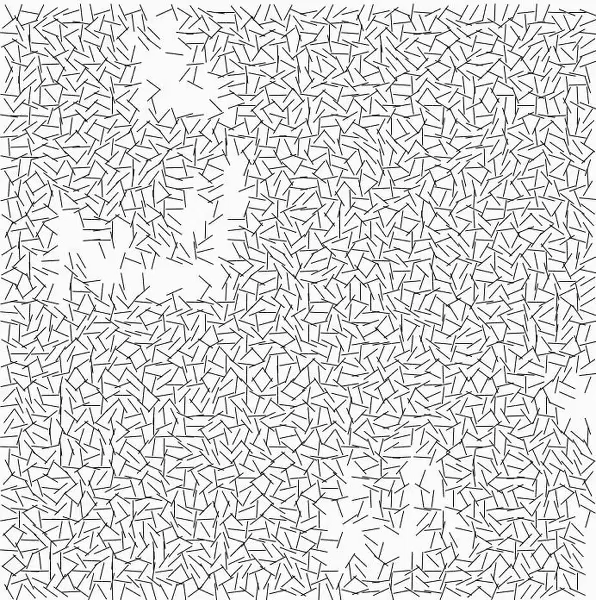# chaine-Interruptions

1.  The lines' centers can be split into a grid system
2.  All angles are randomized
3.  White or light grayish background with black lines
4. The overall shape of the canvas is a square but some lines may protrude a bit more
5.  There are clutters of gaps that seem to happen in random locations
6.  The gaps are usually clumped together and are missing more than one line per gap
7.  Each gap seem to form into random shapes (not just a circle, square, etc.)
8.  The gaps are of varying sizes
9.  The length of each line is the same
10.  There is a very small margin surrounding the lines```var boolDoRefresh; var size = 40; var gridSpacing = 12; var lineLength = 20; var angleList = []; var startAngle = 0;   //Things to consider while creating "gaps": //how many gaps? //how large are the gaps? //which row/col are the gaps located?   var gapList = [];   function setup(){ createCanvas(720,720); boolDoRefresh = true; var slant = random(0,1); var isVertical = random(0,1); for (var i = 0; i &lt; width + lineLength; i += gridSpacing){ for (var j = 0; j &lt; height + lineLength; j += gridSpacing){ if (isVertical &lt; 0.5){ append(angleList, random(-1*PI, PI)); //angle of line horizontal } else{ append(angleList, random(-1*PI, PI) + (PI)); //angle of line vertical } } } createGaps(); }   function createGaps(){ var gridSlots = sq(round(((width + lineLength + 1)/gridSpacing))) var numGap = int(random(3,15)); for (var k = 0; k < numGap; k += 1){ gapList = append(gapList, int(random(1, gridSlots))); gapSize = int(random(10,80)); for (var m = 1; m < gapSize; m += 1){ chance = random(0,1); if (random(0,1) < 0.8){ if (chance < 0.4){ append(gapList, gapList[m-1] + 1); } else{ append(gapList, gapList[m-1] - 1); } } else{ if (chance < 0.6){ append(gapList, gapList[m-1] + 59); } else{ append(gapList, gapList[m-1] - 59); } } for (var n = 0; n < gapList.length; n += 1){ angleList[gapList[n]] = 1000; } } gapList = []; } }   function draw(){ if (boolDoRefresh){ for (var i = lineLength; i &lt; width - lineLength; i += gridSpacing){ for (var j = lineLength; j &lt; height - lineLength; j += gridSpacing){ strokeWeight(1); //ellipse(i,j, 5,5); var angle = angleList[startAngle]; if (angle &lt; 999){ //I gave each line their own little "squares" on a grid of W x H //Each grid consists of two lines, one line is a reflection of the other line //Both lines start at the center of their "squares" //Thus, the two lines together look like one line line(i, j , i+(lineLength/2)*cos(angle), j+(lineLength/2)*sin(angle)); line(i, j , i+(lineLength/2)*cos(angle+PI), j+(lineLength/2)*sin(angle+PI)); } startAngle += 1; } } } boolDoRefresh = false; }   function mousePressed(){ boolDoRefresh = true; angleList = []; startAngle = 0; gapList = []; setup(); }```

I started this project by realizing that there was some sort of grid system with a randomized, angled line in each grid unit. I first decided to code the overall grid without any gaps, but realized that too many of the lines started to look like they were forming messy clusters. (e.g. one grid unit had a line pointing to the right and the very next grid unit to the right was pointing left and it just looked like two lines on top of each other) Therefore, I decided to give each line their own square "space" by drawing two lines to look like one. I first drew half of a line starting from the center of a grid unit and reflected it to draw the other half. That worked out much better. Meanwhile, for randomizing the gaps, I sketched out what I needed to know: how many gaps, how large each gap is, where the gap is. I learned that what I thought was random was clearly showing that it didn't feel as random in real life. There were sometimes columns of missing lines and I struggled to find the right proportion of hiding the aforementioned patterns. I learned through this that coding was a test of trial and error and logic. What may seem logically sound on paper may not look it in the actual product. The fact that Vera Molnár created this piece fascinated and inspired me, especially given the time period in which she created this.